Search

Complex Numbers & The Quadratic Formula (page 3 of 3)

Sections: Introduction, Operations with complexes, The Quadratic FormulaYou'll probably only use complexes in the context of solving quadratics for their zeroes. (There are many other practical uses for complexes, but you'll have to wait for more interesting classes like "Engineering 201" to get to the "good stuff".)

Remember that the Quadratic Formula solves "ax2 + bx + c = 0" for the values of x. Also remember that this means that you are trying to find the x-intercepts of the graph. When the Formula gives you a negative inside the square root, you can now simplify that zero by using complex numbers. The answer you come up with is a valid "zero" or "root" or "solution" for "ax2 + bx + c = 0", because, if you plug it back into the quadratic, you'll get zero after you simplify. But you cannot graph a complex number on the x,y-plane. So this "solution to the equation" is not an x-intercept. You can make this connection between the Quadratic Formula, complex numbers, and graphing:

 x2  2x  3 x2  6x + 9 x2 + 3x + 3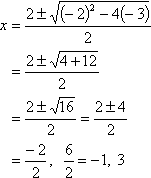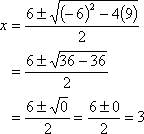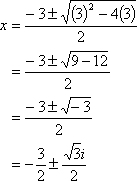a positive number inside the square root zero inside the square root a negative number inside the square root two real solutions one (repeated) real solution two complex solutions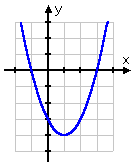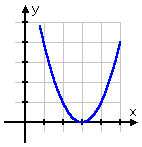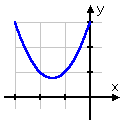two distinct x-intercepts one (repeated) x-intercept no x-intercepts

As an aside, you can graph complexes, but not in the x,y-plane. You need the "complex" plane. For the complex plane, the x-axis is where you plot the real part, and the y-axis is where you graph the imaginary part. For instance, you would plot the complex number 3  2i like this: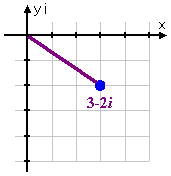This leads to an interesting fact: When you learned about regular ("real") numbers, you also learned about their order (this is what you show on the number line). But x,y-points don't come in any particular order. You can't say that one point "comes after" another point in the same way that you can say that one number comes after another number. For instance, you can't say that (4, 5) "comes after" (4, 3) in the way that you can say that 5 comes after 3. Pretty much all you can do is compare "size", and, for complex numbers, "size" means "how far from the origin". To do this, you use the Distance Formula, and compare which complexes are closer to or further from the origin. This "size" concept is called "the modulus". For instance, looking at our complex number plotted above, its modulus is computed by using the Distance Formula:   Copyright © Elizabeth Stapel 2000-2011 All Rights Reserved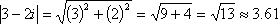Note that all points at this distance from the origin have the same modulus. All the points on the circle with radius sqrt(13) are viewed as being complex numbers having the same "size" as 3  2i.

<< Previous  Top  |  1 | 2 | 3  |  Return to Index

 Cite this article as: Stapel, Elizabeth. "Complex Numbers & The Quadratic Formula." Purplemath. Available from     https://www.purplemath.com/modules/complex3.htm. Accessed [Date] [Month] 2016

Study Skills Survey

Tutoring from Purplemath
Find a local math tutor